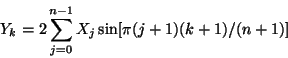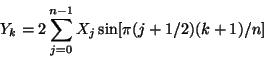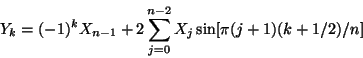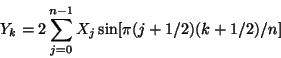Next: , Previous: , Up: What FFTW Really Computes   [Contents][Index]

#### 4.8.4 1d Real-odd DFTs (DSTs)

The Real-odd symmetry DFTs in FFTW are exactly equivalent to the unnormalized forward (and backward) DFTs as defined above, where the input array X of length N is purely real and is also odd symmetry. In this case, the output is odd symmetry and purely imaginary.

For the case of `RODFT00`, this odd symmetry means that Xj = -XN-j, where we take X to be periodic so that XN = X0. Because of this redundancy, only the first n real numbers starting at j=1 are actually stored (the j=0 element is zero), where N = 2(n+1).

The proper definition of odd symmetry for `RODFT10`, `RODFT01`, and `RODFT11` transforms is somewhat more intricate because of the shifts by 1/2 of the input and/or output, although the corresponding boundary conditions are given in Real even/odd DFTs (cosine/sine transforms). Because of the odd symmetry, however, the cosine terms in the DFT all cancel and the remaining sine terms are written explicitly below. This formulation often leads people to call such a transform a discrete sine transform (DST), although it is really just a special case of the DFT.

In each of the definitions below, we transform a real array X of length n to a real array Y of length n:

#### RODFT00 (DST-I)

An `RODFT00` transform (type-I DST) in FFTW is defined by:.

#### RODFT10 (DST-II)

An `RODFT10` transform (type-II DST) in FFTW is defined by:.

#### RODFT01 (DST-III)

An `RODFT01` transform (type-III DST) in FFTW is defined by:.
In the case of n=1, this reduces to Y0 = X0.

#### RODFT11 (DST-IV)

An `RODFT11` transform (type-IV DST) in FFTW is defined by:.

#### Inverses and Normalization

These definitions correspond directly to the unnormalized DFTs used elsewhere in FFTW (hence the factors of 2 in front of the summations). The unnormalized inverse of `RODFT00` is `RODFT00`, of `RODFT10` is `RODFT01` and vice versa, and of `RODFT11` is `RODFT11`. Each unnormalized inverse results in the original array multiplied by N, where N is the logical DFT size. For `RODFT00`, N=2(n+1); otherwise, N=2n.

In defining the discrete sine transform, some authors also include additional factors of √2 (or its inverse) multiplying selected inputs and/or outputs. This is a mostly cosmetic change that makes the transform orthogonal, but sacrifices the direct equivalence to an antisymmetric DFT.

Next: , Previous: , Up: What FFTW Really Computes   [Contents][Index]